# Calculating Forces Required to Cause Prescribed Motion of a Particle (Part - 2) Civil Engineering (CE) Notes | EduRev

## Civil Engineering (CE) : Calculating Forces Required to Cause Prescribed Motion of a Particle (Part - 2) Civil Engineering (CE) Notes | EduRev

The document Calculating Forces Required to Cause Prescribed Motion of a Particle (Part - 2) Civil Engineering (CE) Notes | EduRev is a part of the Civil Engineering (CE) Course Introduction to Dynamics and Vibrations- Notes, Videos, MCQs.
All you need of Civil Engineering (CE) at this link: Civil Engineering (CE)

Alternative method of solution using normal-tangential coordinates

We will solve this problem again, but this time we’ll use the short-cuts described in Section 3.1.4 to write down the acceleration vector, and we’ll write down the vectors in Newton’s laws of motion in terms of the unit vectors n and t normal and tangent to the object’s path.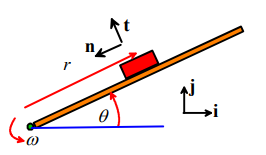(i) Acceleration vector If the block does not slip, it moves with speed V = ωr around a circular arc with radius r. Its acceleration vector has magnitude V2 / r and direction parallel to the unit vector n.

(ii) The force vector can be resolved into components parallel to n and t. Simple trig on the free body diagram shows that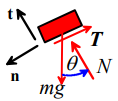F = (N - mg cosθ) t + (mg sinθ - T) n = mω2rn

The components of this vector equation parallel to t and n yield two equations, with solution

N = mg cosθ T = mg sinθ - mω2r

This is the same solution as before. The short-cut makes the calculation slightly more straightforward. This is the main purpose of using normal-tangential components.

Example 6: Window blinds. Have you ever wondered how window shades work? You give the shade a little downward jerk, let it go, and it winds itself up. If you pull the shade down slowly, it stays down.

The figure shows the mechanism (which probably only costs a few cents to manufacture) that achieves this remarkable feat of engineering. It’s called an `inertial latch’ – the same principle is used in the inertia reels on the seatbelts in your car.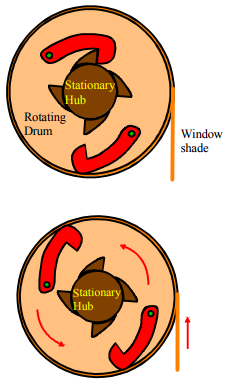The picture shows an enlarged end view of the window shade. The hub, shown in brown, is fixed to the bracket supporting the shade and cannot rotate. The drum, shown in peach, rotates as the shade is pulled up or down. The drum is attached to a torsional spring, which tends to cause the drum to rotate counterclockwise, so winding up the shade. The rotation is prevented by the small dogs, shown in red, which engage with the teeth on the hub. You can pull the shade downwards freely, since the dogs allow the drum to rotate counterclockwise.

To raise the shade, you need to give the end of the shade a jerk downwards, and then release it. When the drum rotates sufficiently quickly (we will calculate how quickly shortly) the dogs open up, as shown on the right. They remain open until the drum slows down, at which point the topmost dog drops and engages with the teeth on the hub, thereby locking up the shade once more.

We will estimate the critical rotation rate required to free the rotating drum.

1. Idealization – We will idealize the topmost dog as a particle on the end of a massless, inextensible rod, as shown in the figure.

a. We will assume that the drum rotates at constant angular rate ω . Our goal is to calculate the critical speed where the dog is just on the point of dropping down to engage with the hub.

b. When the drum spins fast, the particle is contacts the outer rim of the drum – a normal force acts at the contact. When the dog is on the point of dropping this contact force goes to zero. So our goal is to calculate the contact force, and then to find the critical rotation rate where the force will drop to zero.

c. We neglect friction.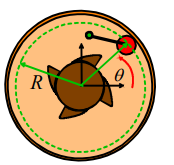2. FBD. The figure shows a free body diagram for the particle. The particle is subjected to: (i) a reaction force N where it contacts the rim; (ii) a tension T in the link, and (iii) gravity. The resultant force is

F = -T cos(φ -θ) - N cosθ) i + (- N sinθ + T sin(φ -θ) - mg) j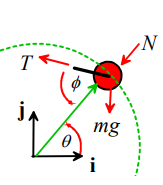3. Kinematics We can use the circular motion formula to write down the acceleration of the particle(see section 3.1.3)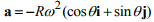4. EOM: The equation of motion is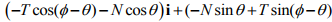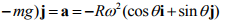5. Solution: The i and j components of the equation of motion can be solved for N and T – Mupad makes this painless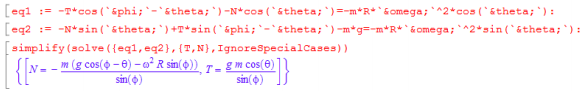normal reaction force is therefore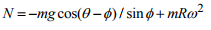We are looking for the point where this can first become zero or negative. Note that max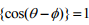at the point where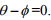The smallest value of N therefore occurs at this point, and has magnitude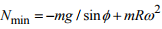The critical speed where N=0 follows as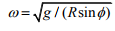Changing the angle φ and the radius R gives a convenient way to control the critical speed in designing an inertial latch.

Alternative solution using polar coordinates

We’ll work through the same problem again, but this time handle the vectors using polar coordinates.

1. FBD. The figure shows a free body diagram for the particle. The particle is subjected to: (i) a reaction force N where it contacts the rim; (ii) a tension T in the link, and (iii) gravity. The resultant force is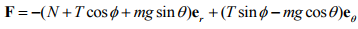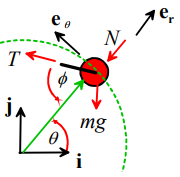2. Kinematics The acceleration vector is now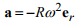3. EOM: The equation of motion is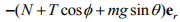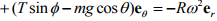4. Solution: The , er, eθ components of the equation of motion can be solved for N and T – again, we can use Mupad for this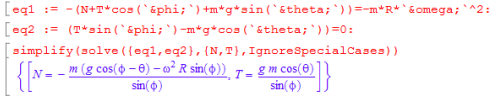The normal reaction force is therefore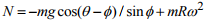We are looking for the point where this can first become zero or negative. Note that max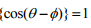at the point where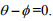The smallest value of N therefore occurs at this point, and has magnitude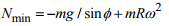The critical speed where N=0 follows as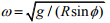Changing the angle φ and the radius R gives a convenient way to control the critical speed in designing an inertial latch.

Example 7: Aircraft Dynamics Aircraft performing certain instrument approach procedures (such as holding patterns or procedure turns) are required to make all turns at a standard rate, so that a complete 360 degree turn takes 2 minutes. All turns must be made at constant altitude and constant speed, V.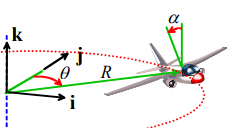People who design instrument approach procedures need to know the radius of the resulting turn, to make sure the aircraft won’t hit anything. Engineers designing the aircraft are interested in the forces needed to complete the turn – specifically, the load factor, which is the ratio of the lift force on the aircraft to its weight.

In this problem we will calculate the radius of the turn R and the bank angle required, as well as the load factor caused by the maneuver, as a function of the aircraft speed V.

Before starting the calculation, it is helpful to understand what makes an aircraft travel in a circular path. Recall that

1. If an object travels at constant speed around a circle, its acceleration vector has constant magnitude, and has direction towards the center of the circle
2. A force must act on the aircraft to produce this acceleration – i.e. the resultant force on the aircraft must act towards the center of the circle. The necessary force comes from the horizontal component of the lift force – the pilot banks the wings, so that the lift acts at an angle to the vertical.

With this insight, we expect to be able to use the equations of motion to calculate the forces.

1. Idealization – The aircraft is idealized as a particle – it’s not obvious that this is accurate, because the aircraft clearly rotates as it travels around the curve. However, the forces we wish to calculate turn out to be fully determined by F=ma and are not influenced by the rotational motion.

2. FBD. The figure shows a free body diagram for the aircraft. It is subjected to (i) a gravitational force (mg); (ii) a thrust from the engines FT , (iii) a drag force FD , acting perpendicular to the direction of motion, and (iv) a lift force FL , acting perpendicular to the plane of the wings

The resultant force is

[(FT —FD)cosθ — FL sinα sinθ]i + [(FD -FT)sinθ —FL sinα cosθ] j+ {FL cosα— mg)k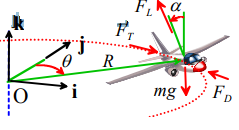(you may find the components of the lift force difficult to visualize – to see where these come from, note that the lift force can be projected onto components along OR and the k direction as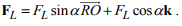Then note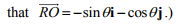3. Kinematics

a. The aircraft moves at constant speed around a circle, so the angle θ = ωt, where ω is the (constant) angular speed of the line OP. Since the aircraft completes a turn in two minutes, we know that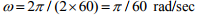b. The position vector of the plane is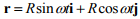We can differentiate this expression with respect to time to find the velocity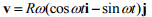c. The magnitude of the velocity is V = Rω, so if the aircraft flies at speed V, the radius of the turn must be R = V/ω

d. Differentiating the velocity gives the acceleration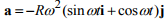4. EOM: The equation of motion is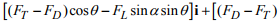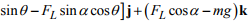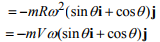5. Solution: The i j and k components of the equation of motion give three equations that can be solved for FT , FL and α . We assume that the drag force is known, since this is a function of the aircraft’s speed.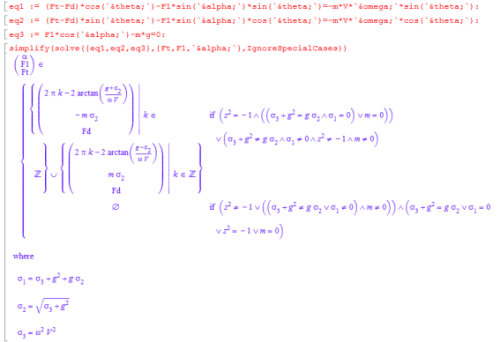This solution is correct, but you need a PhD to understand what it means (other symbolic manipulation programs like Maple and Mathematica give a comprehensible solution). Fortunately, I happen to have a PhD… The solution can be simplified to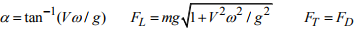As we will see below, if you choose to solve this problem in normal-tangential coordinates, you don’t need a PhD to

We can calculate values of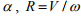and the load factor FL / mg for a few aircraft

a. Cessna 150 – V=70knots (36 m/s) : α = 110 R=690m, FL / mg = 1.02

b. Boeing 747: V=200 knots (102 m/s) α = 280 R=1950m, FL / mg = 1.014

c. F111 V=300 knots (154 m/s) α = 390  R=2950m, FL / mg = 1.3

Alternative solution using normal-tangential coordinates

This problem can also be solved rather more quickly using normal and tangential basis vectors.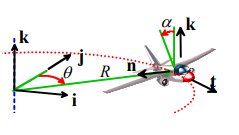(i) Acceleration vector. The aircraft travels around a circular path at constant speed, so its acceleration is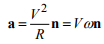where n is a unit vector pointing towards the center of the circle.

(ii) Force vector. The force vector can be written in terms of the unit vectors n,t,k as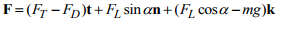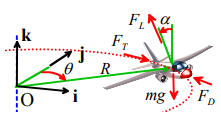(iii) Newton’s law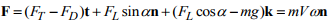The n, t and k components of this equation give three equations that can be solved for FT , FL and α . This time it is easy to solve the equations by hand…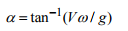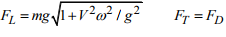This example again shows why normal-tangential coordinates are useful – describing forces, and solving the resulting force-acceleration relations are much simpler than working with a fixed coordinate system.

Offer running on EduRev: Apply code STAYHOME200 to get INR 200 off on our premium plan EduRev Infinity!

## Introduction to Dynamics and Vibrations- Notes, Videos, MCQs

20 videos|53 docs

,

,

,

,

,

,

,

,

,

,

,

,

,

,

,

,

,

,

,

,

,

;